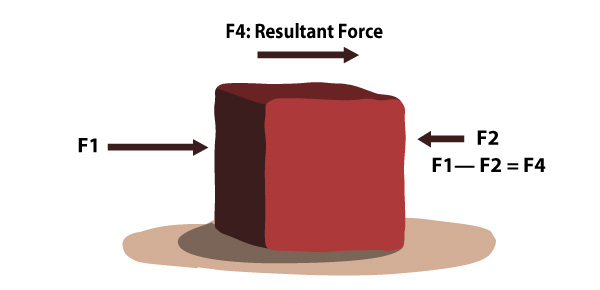## Resultant forces

To understand resultant forces better, let us see these two scenarios:

Any time a stationary object stays still, its resultant force is zero. As soon as force is applied, acceleration begins. The speed of the acceleration will depend on the force applied and the mass of the object.

Similarly, each time an object in motion (in a constant speed and same direction) stays in motion, its resultant force is zero too. As soon as a force is applied, it can make it stop, change direction, move slower or move faster. The effect will depend on the force applied and the mass of the object.

It is worth noting that an object may have several different forces acting on it. See the example in the illustration below:All these different forces, F1, F2, F3 can be added up to know the resultant force, F4. The resultant force is the single force that has the same effect on the object as all the individual forces acting together.

If different forces are acting in different directions, a resultant force can be determined as well. See the illustration below:##### Notes

If the resultant force acting on a stationary object is zero, the object will remain stationary.

If the resultant force acting on a moving object is zero, the object will carry on moving at the same speed in the same direction (i.e. at constant velocity).